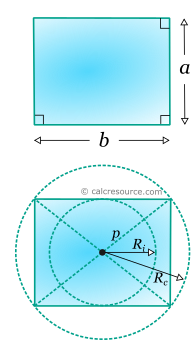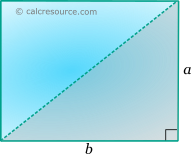## Properties of a Rectangle

This tool calculates the basic geometric properties of a rectangle. Enter below the shape dimensions. The calculated results will have the same units as your input. Please use consistent units for any input.

 a = b = Geometric properties: Area = Perimeter = Diagonal p = Inradius Ri = Circumradius Rc =## Definitions

### Geometry

Rectangle is a quadrilateral shape with two pairs of parallel sides, that meet each other at right angles. If all sides are equal the shape is a square. The area of a rectangle is given by the formula:

where a, b the lengths of the sides.

The perimeter of a rectangle is simply the sum of the lengths of all sides:

The length of the diagonal, can be expressed in terms of the length of the sides, using the Pythagorean theorem on the right triangle with the diagonal as hypotenuse: